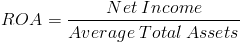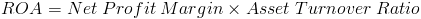# Return on AssetsThe return on assets formula, sometimes abbreviated as ROA, is a company's net income divided by its average of total assets. The return on assets formula looks at the ability of a company to utilize its assets to gain a net profit.

Net income in the numerator of the return on assets formula can be found on a company's income statement. Net income is the amount earned by a company after subtracting out the expenses incurred, including depreciation and taxes.

Average total assets in the denominator of the return on assets formula is found on a company's balance sheet. The average of total assets should be used based on the period being evaluated. For example, if an investor is calculating a company's 2015 return on assets, the beginning and ending total assets for that year should be averaged.

## ROA Formula vs. Asset Turnover Ratio

The distinct difference between return on assets and asset turnover is that the return on assets considers net income and asset turnover considers revenues. By using net income instead of revenues, the return on assets formula factors in a company's expenses.

The asset turnover ratio can be used to calculate return on assets with the following formulaNet Profit Margin is revenues divided by net income and the asset turnover ratio is net income divided average total assets. By multiplying these two together, revenues is cancelled out leaving the formula for return on assets shown on top of the page.

## Use of ROA Formula

The return on assets formula can be used by an investor or by a company internally to evaluate if the company is turning a profit relative to their assets. It is important for an investor to consider that a company's return on assets can vary depending on which industry the company does business in. A particular company may provide a product that requires additional assets to manufacture the product relative to another industry.

New to Finance?